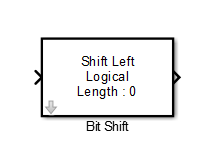# Bit Shift

Logical or arithmetic shift of input signal

•Libraries:
HDL Coder / Logic and Bit Operations

## Description

The Bit Shift block performs a logical or arithmetic shift on the input signal.

This block is different from the Shift Arithmetic block in terms of simulation and HDL code generation behavior. The Bit Shift block can perform logical shifting of a signed number without having to perform a `reinterpretcast` operation. This block uses a MATLAB Function block based implementation and might be slower in operation.

The Shift Arithmetic block shifts the bits or binary point of the input number. This block has additional block options for HDL code generation in comparison to the Bit Shift block. When you want to perform a variable shift operation, use the Shift Arithmetic block instead of the Bit Shift block. If you have a signed number as input, the block performs a sign extension of the number. The Shift Arithmetic block requires using additional Data Type Conversion blocks that have the ```Stored Integer (SI)``` option selected.

## Ports

### Input

expand all

Input signal on which the logical or arithmetic shift is performed.

• Minimum bit width: 2

• Maximum bit width: 128

Data Types: `single` | `double` | `int8` | `int16` | `int32` | `int64` | `uint8` | `uint16` | `uint32` | `uint64` | `Boolean` | `fixed point`

### Output

expand all

Output signal that is logically or arithmetically shifted. The output signal has the same data type and bit width as the input signal.

• Minimum bit width: 2

• Maximum bit width: 128

## Parameters

expand all

Specifies the type and direction of shift.

#### Programmatic Use

 Block parameter: `mode` Type: string scalar | character vector Value: `"Shift Left Logical"` | ```"Shift Right Logical"``` | ```"Shift Right Arithmetic"``` Default: `'Shift Left Logical'`

Specifies the number of bits to be shifted. Specify a value greater than or equal to zero.

#### Programmatic Use

 Block parameter: `N` Type: string scalar | character vector Value: A value greater than or equal to zero Default: `'0'`

## Version History

Introduced in R2014a